FEATool Multiphysics  v1.15.5 Finite Element Analysis Toolbox
Black-Scholes Equation

This is an example showing how to define a custom PDE equation model in the FEATool Multiphysics. In this case the Black-Scholes model equation, which is used in financial analytics to model derivatives and options pricing. The non-linear partial differential equation to be solved reads

$\frac{\partial u}{\partial t} - \frac{\partial}{\partial x}(\frac{1}{2}\frac{\partial u}{\partial x}) - \frac{\partial u}{\partial x} = -u + ( (x-t)^5 - 10(x-t)^4 - 10(x-t)^3)$

with boundary conditions u(0,t) = -t^5 and u(x,t) = (x-t)^5 on the left and right sides of the domain, respectively. The problem is time-dependent with initial condition is u(x,t=0) = x^5. For this problem an exact analytical solution exists, u(x,t) = (x-t)^5, which can be used to verify the computed solution. See the Custom Equation section for details on the equation syntax.

# Tutorial

This model is available as an automated tutorial by selecting Model Examples and Tutorials... > Classic PDE > Black-Scholes Equation from the File menu, viewed as a videotutorial", or alternatively, follow the step-by-step instructions below.

1. To start a new model click the New Model toolbar button, or select New Model... from the File menu.
2. Select the 1D radio button.
3. Select the Custom Equation physics mode from the Select Physics drop-down menu.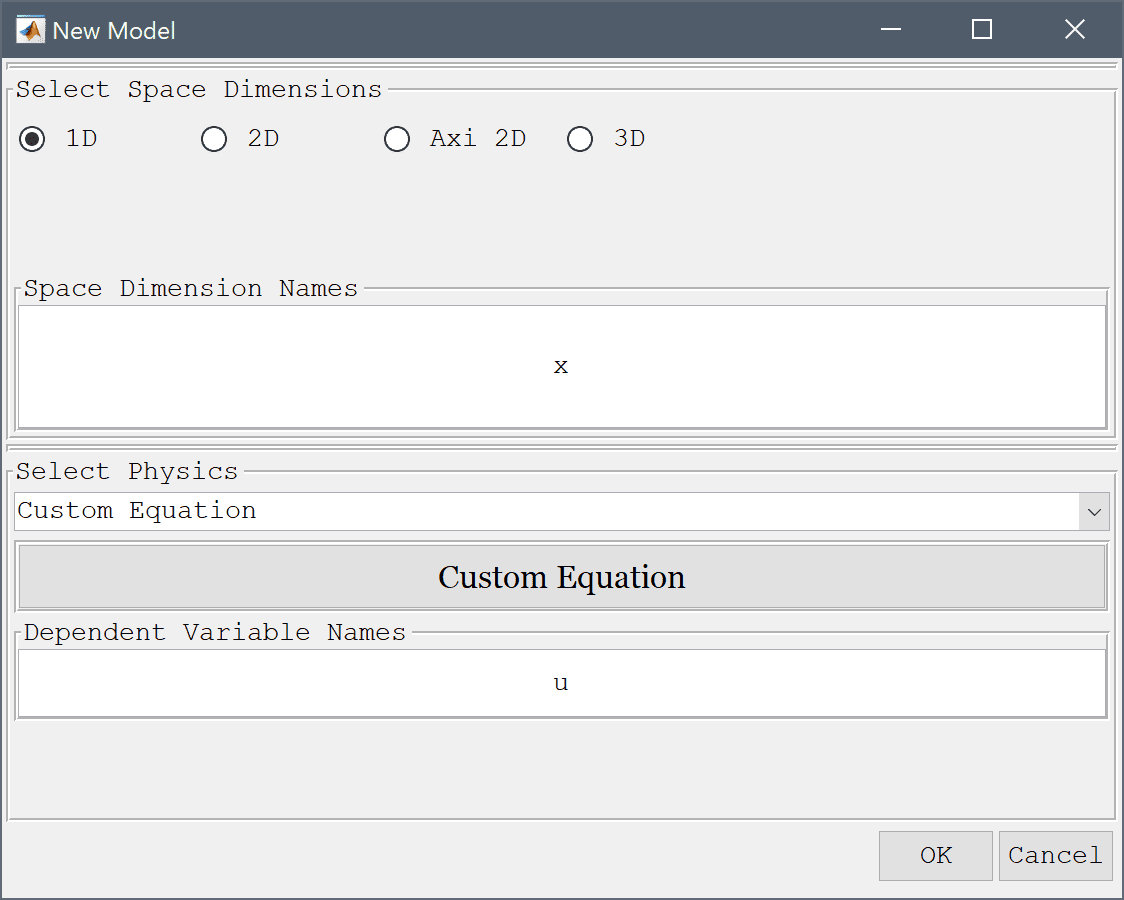4. Press OK to finish the physics mode selection.
5. Press the Create line Toolbar button.
6. Press OK to finish and close the dialog box.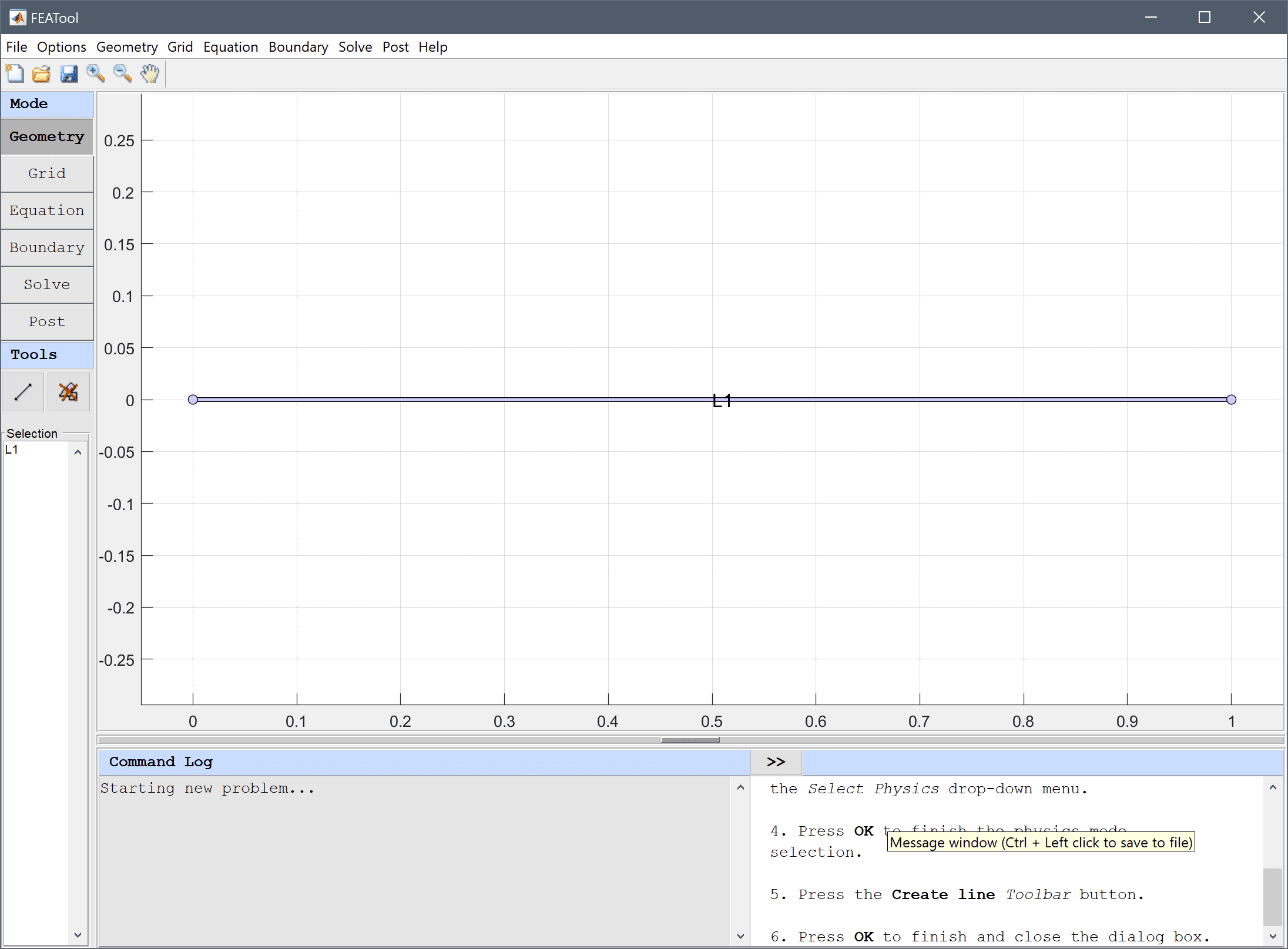7. Switch to Grid mode by clicking on the corresponding Mode Toolbar button.
8. Press the Generate button to call the grid generation algorithm.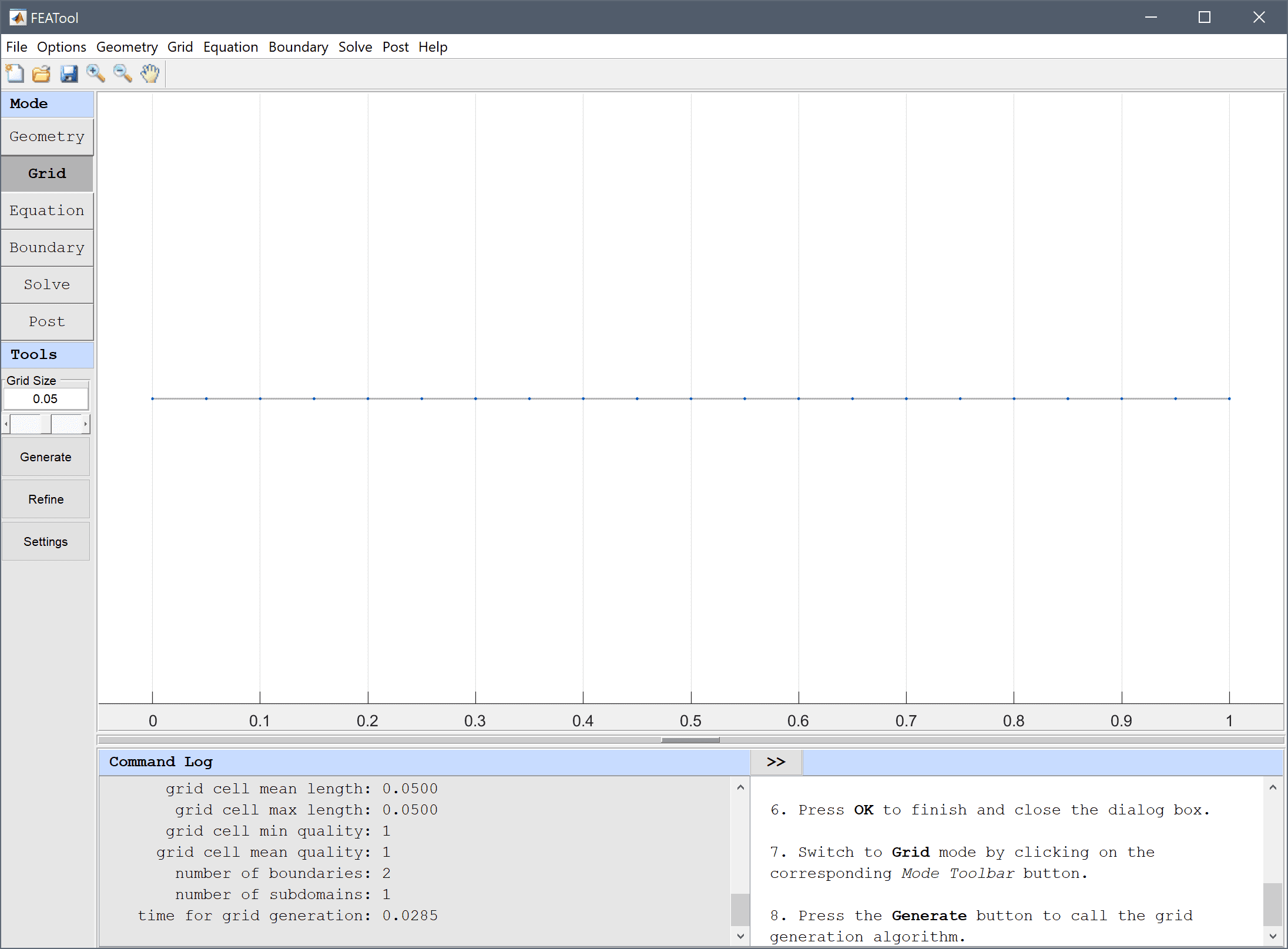9. Switch to Equation mode by clicking on the corresponding Mode Toolbar button.
10. Press the edit button.
11. Enter u' - 1/2*ux_x - ux_t + u_t = (x-t)^5 - 10*(x-t)^4 - 10*(x-t)^3 into the Equation for u edit field.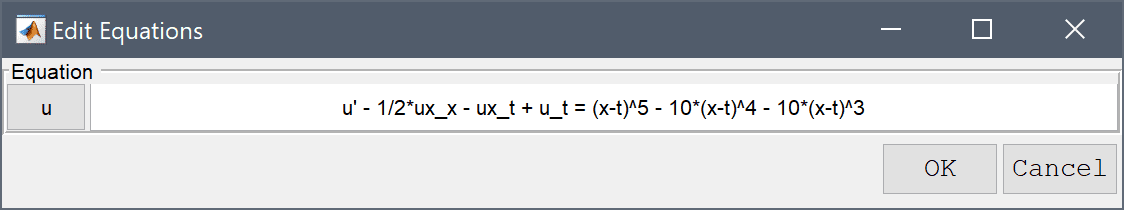12. Press OK to finish and close the dialog box.
13. Enter x^5 into the Initial condition for u edit field.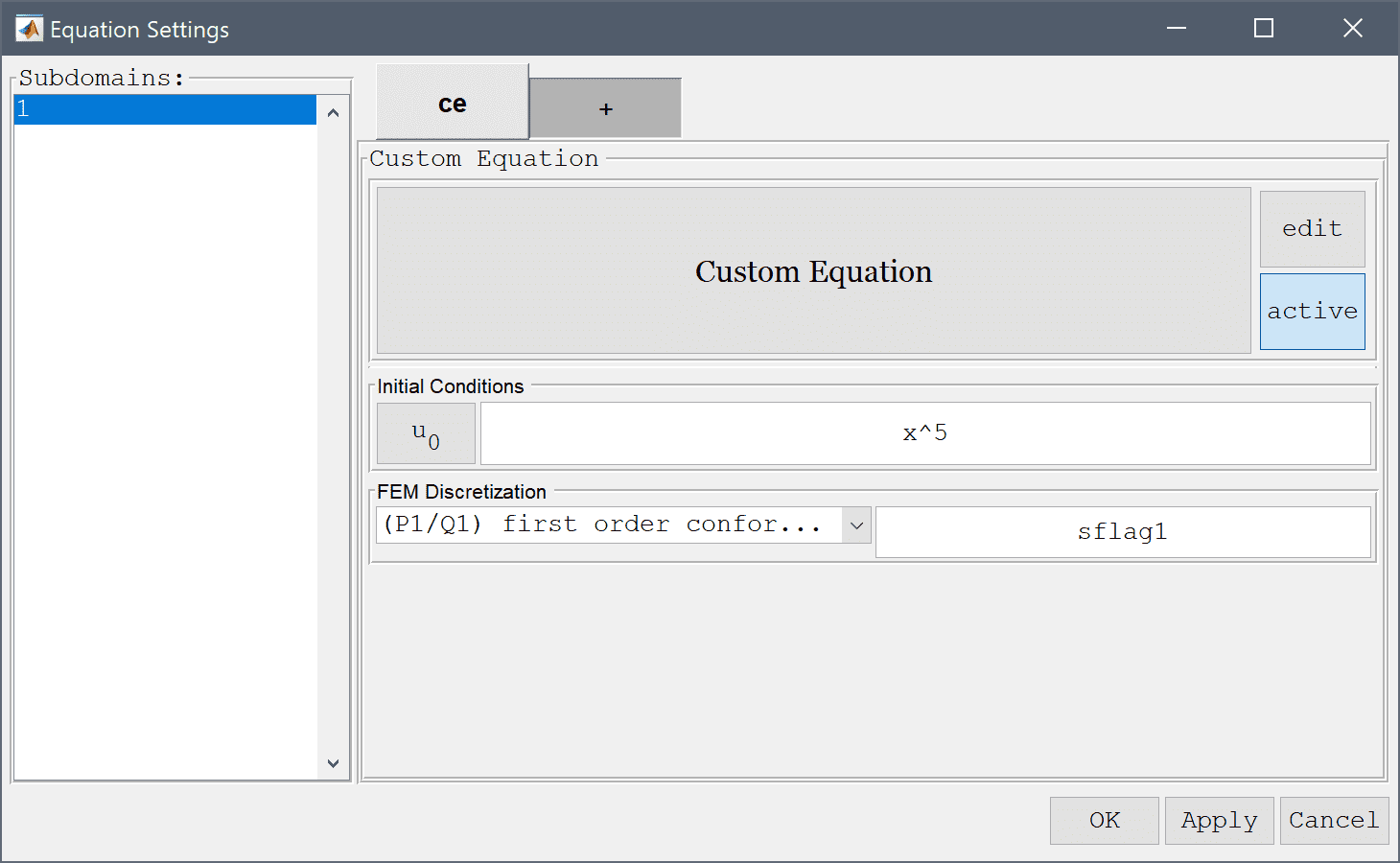14. Press OK to finish the equation and subdomain settings specification.
15. Switch to Boundary mode by clicking on the corresponding Mode Toolbar button.

The values at the two boundaries should be set to -t^5 minus t to the power of 5, at the left boundary, and (1-t)^5 to the right, where t represents time.

1. Enter -t^5 into the Dirichlet/Neumann coefficient edit field.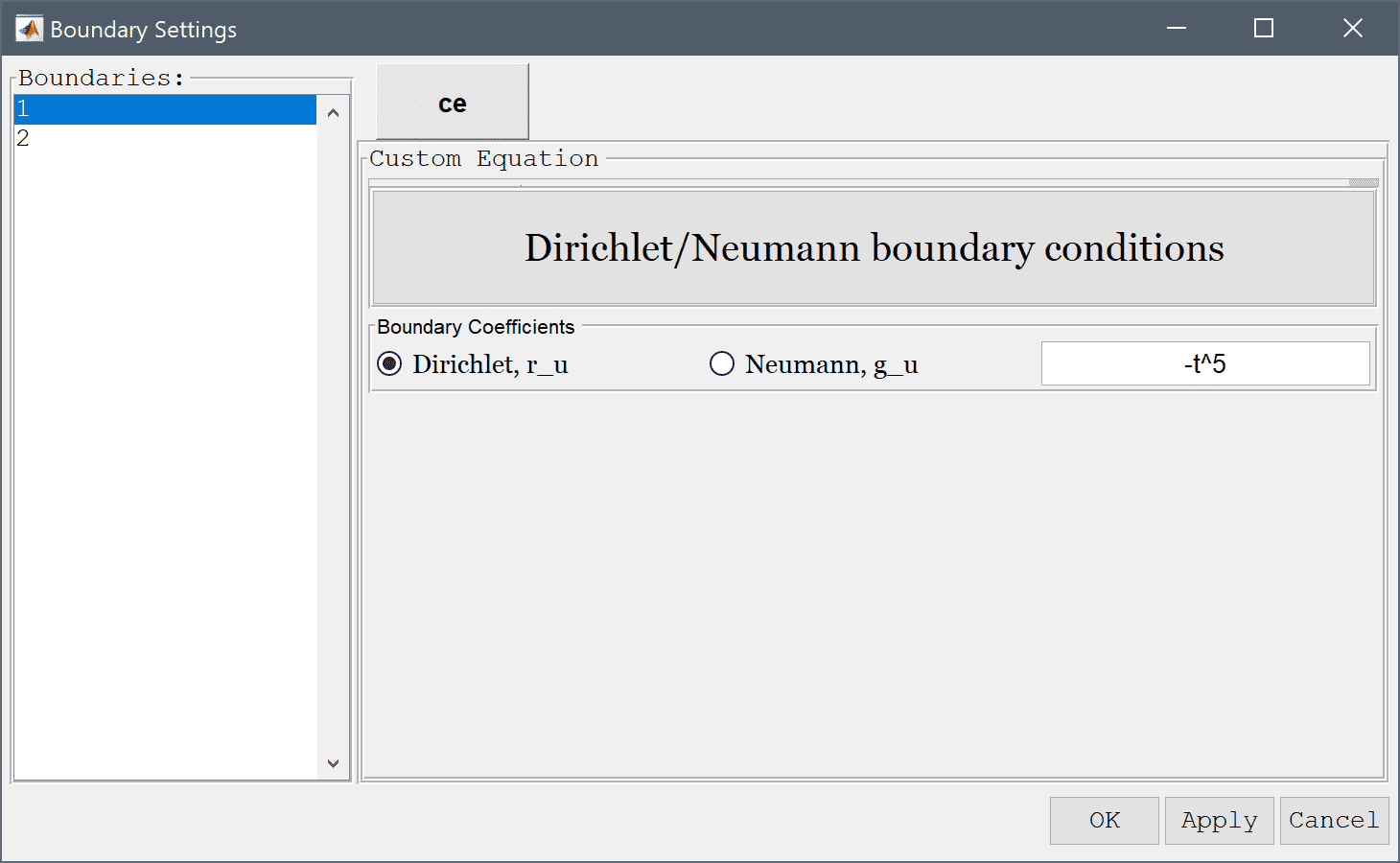2. Select 2 in the Boundaries list box.
3. Enter (1-t)^5 into the Dirichlet/Neumann coefficient edit field.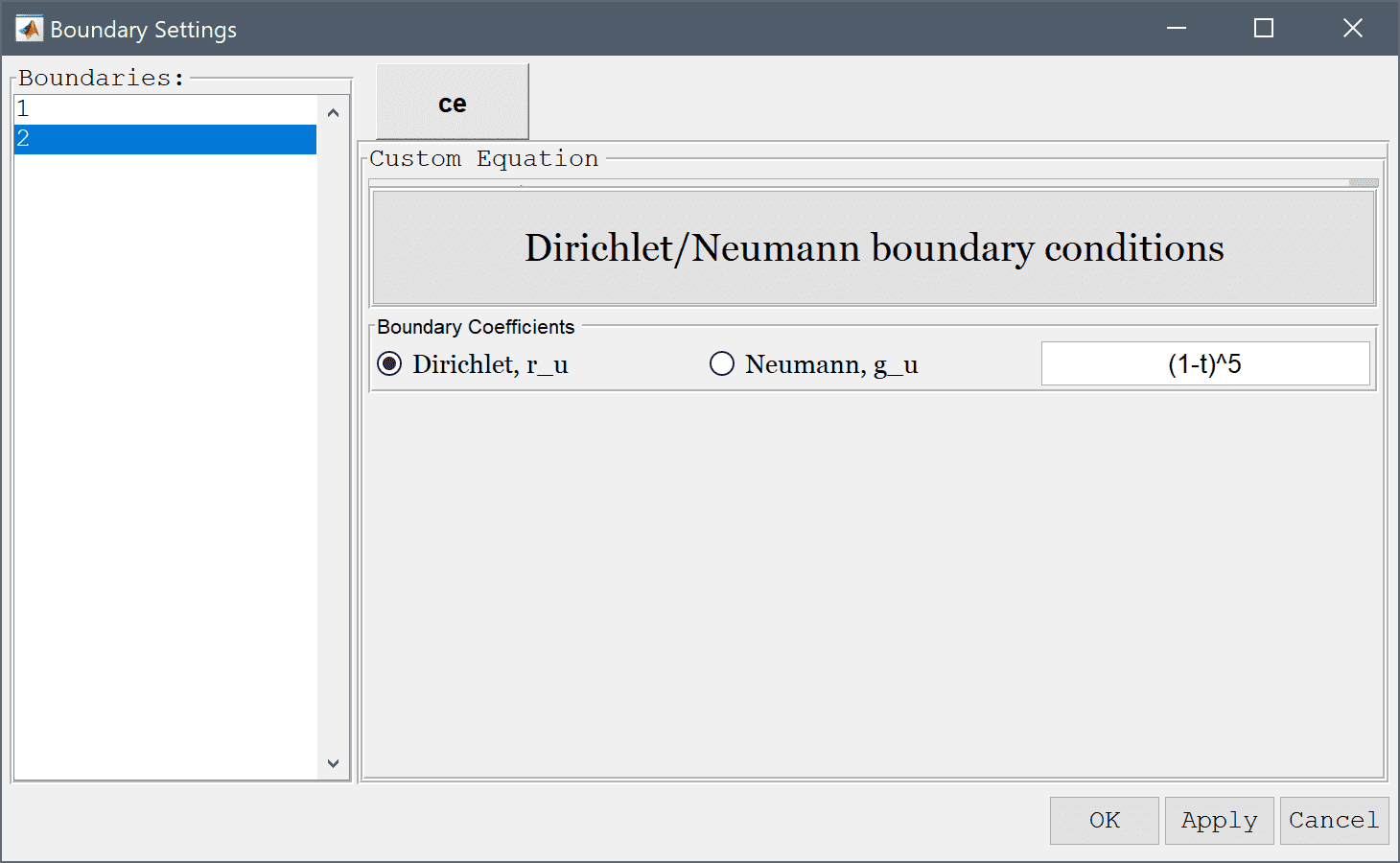4. Press OK to finish the boundary condition specification.
5. Switch to Solve mode by clicking on the corresponding Mode Toolbar button.
6. Press the Settings Toolbar button.
7. Select Time-Dependent from the Solution and solver type drop-down menu.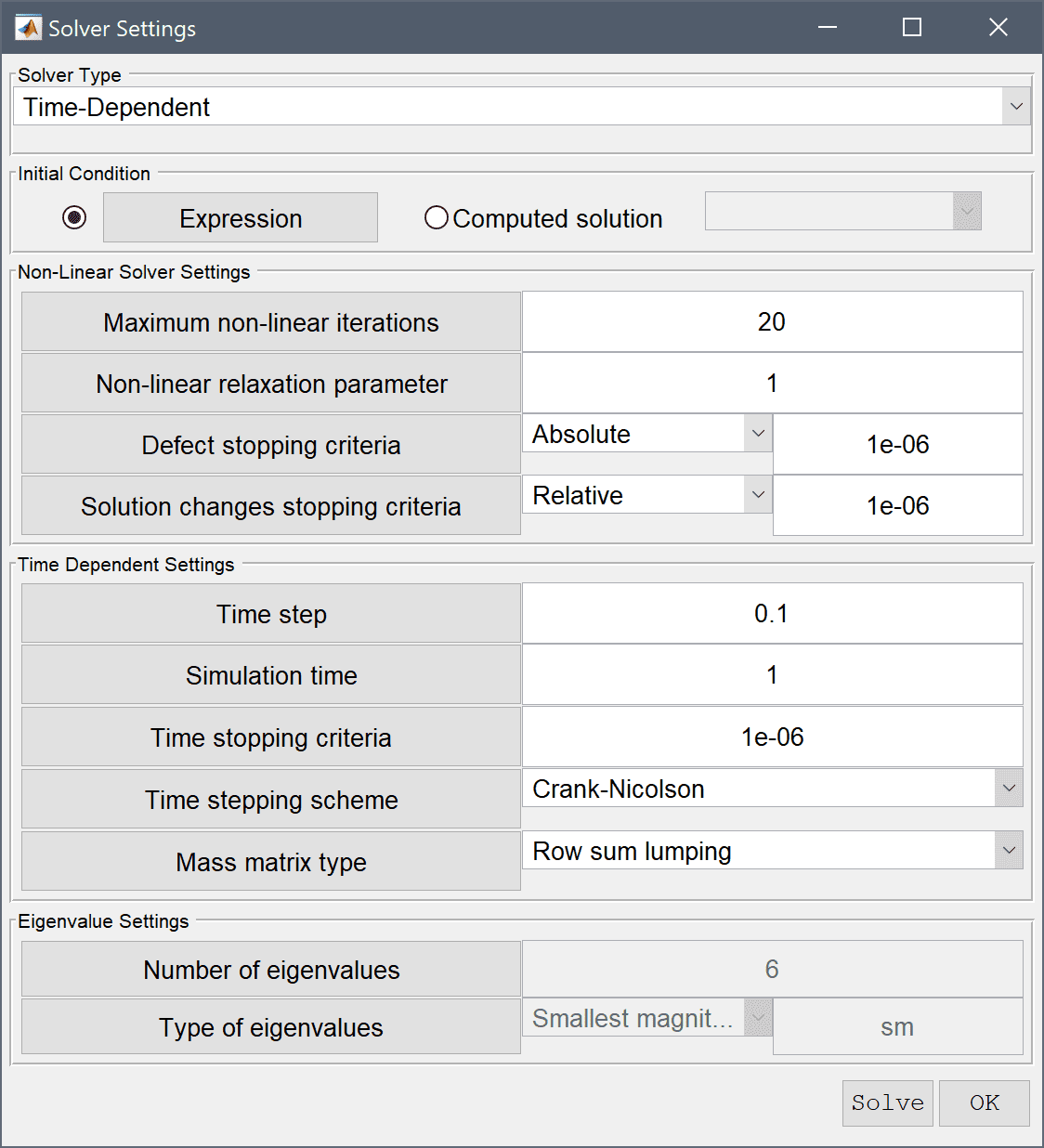8. Press the Solve button.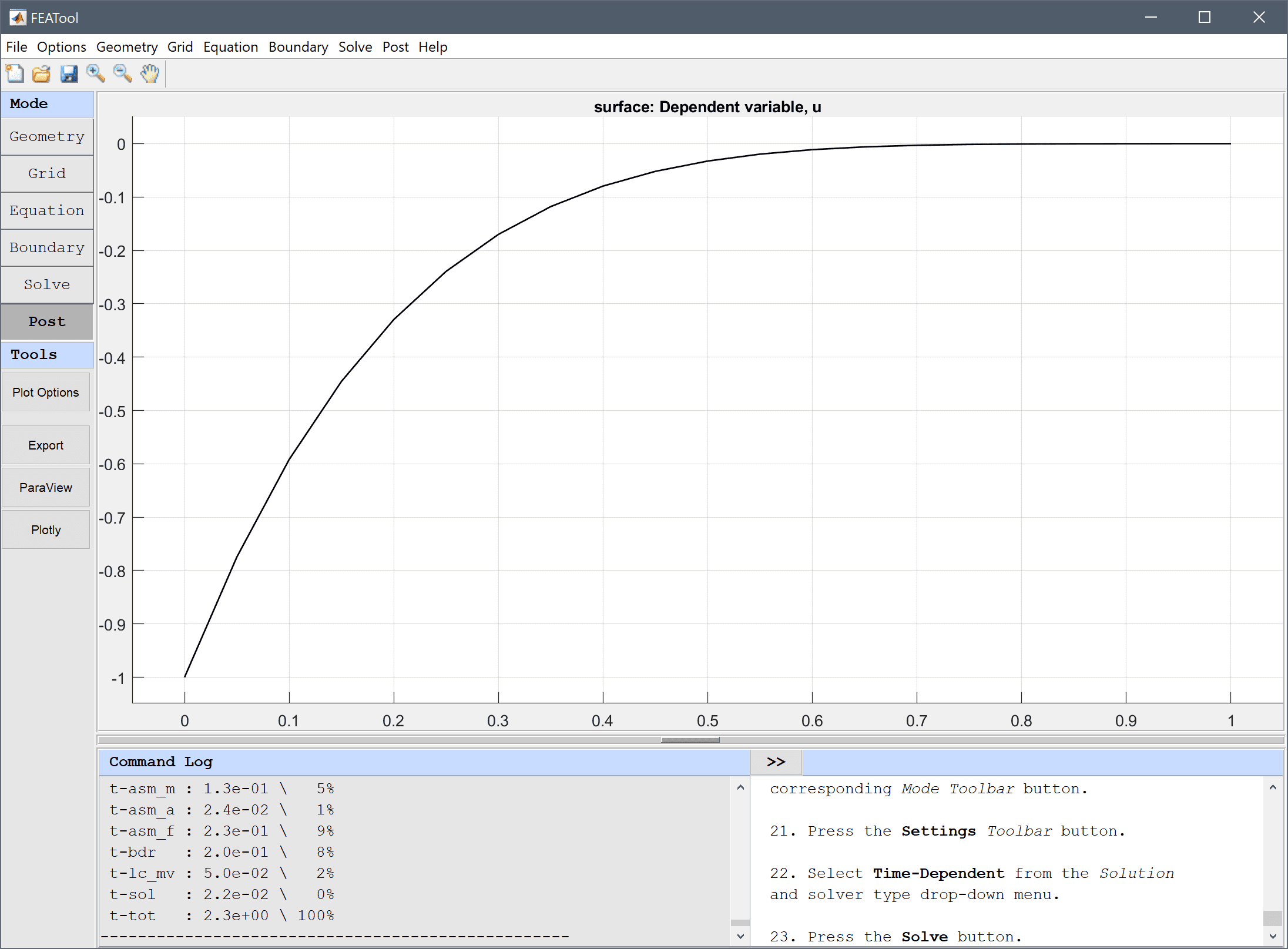9. To visualize the difference between the computed and exact analytical solution, open the Plot Options and postprocessing settings dialog box and enter the expression u - (x-t)^5 in the edit field for the Surface Plot expression.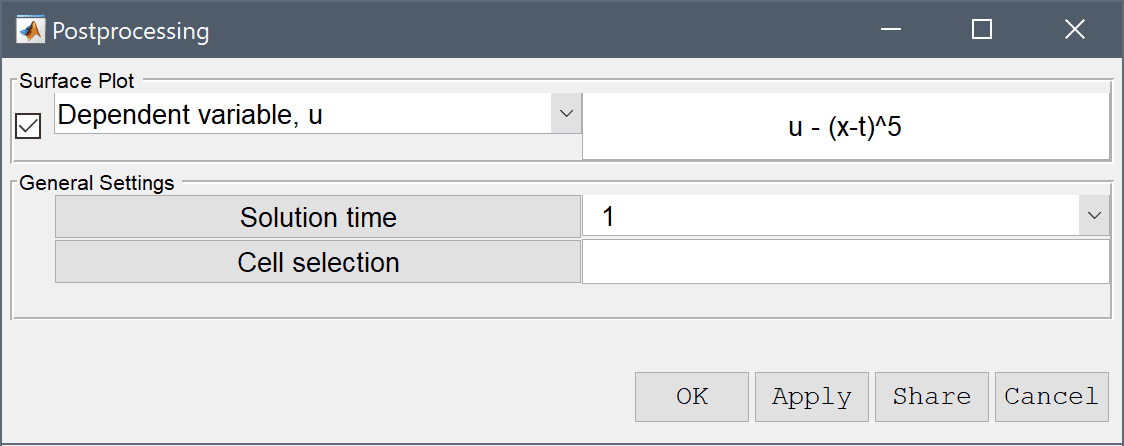10. The magnitude of the error is too small to see clearly with the default axis scaling. Zoom in manually by selecting Axis/Grid Settings... in the Options menu.
11. Select the Axis equal radio button.
12. Clear the Bounding box check box.
13. Select the Axis limits radio button.
14. Enter 0 1 -5e-3 5e-3 into the edit field for the Axis limits (for the xmin, xmax, ymin, and ymax limits).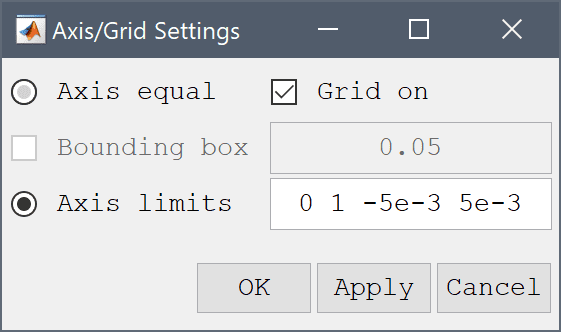15. Press OK to finish and close the dialog box.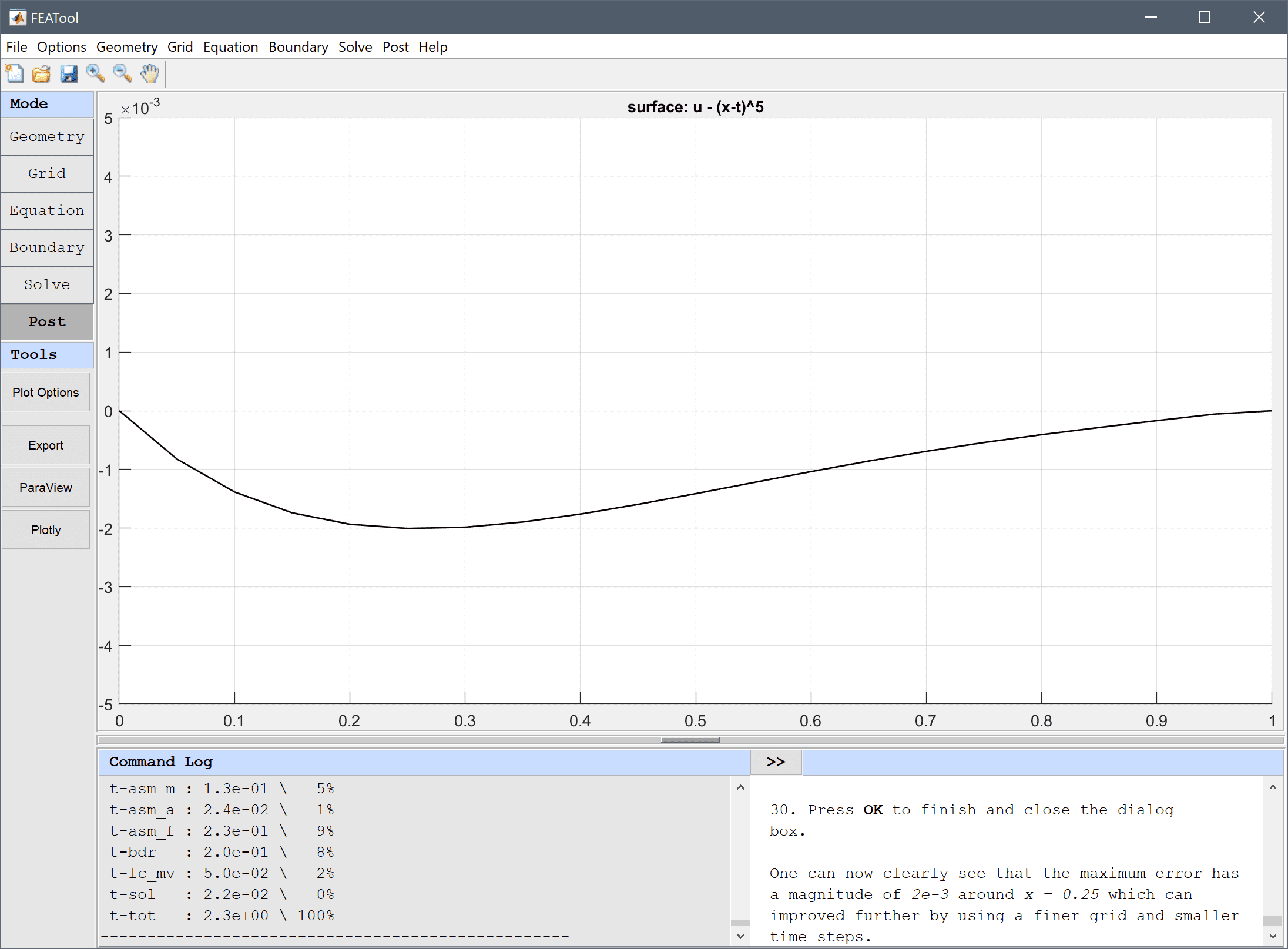One can now clearly see that the maximum error has a magnitude of 0.002 around x = 0.25, which can be improved further by using a finer grid and smaller time steps.

The black-scholes equation classic pde model has now been completed and can be saved as a binary (.fea) model file, or exported as a programmable MATLAB m-script text file (available as the example ex_custom_equation1 script file), or GUI script (.fes) file.